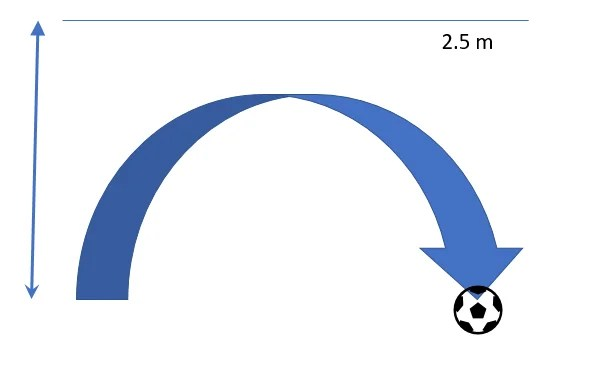Step 1: Substitute  a = -2 and simplify the quadratic equation.

2.5 = -2t2+3t+1

0= -2t2+3t+1-2.5

-2t2+3t-1.5=0

Step 2: Find the discriminant of the equation and show that it is < 0.

(-3)2-4(-2)(-1.5)

9-12<0The ball did not reach a height of 2.5 m. Therefore the flight path of the ball did not intersect the imaginary line 2.5m. Therefore the discriminant will be b2-4ac <0.

Additional Math (A-Math) Group Tuition. Woodlands and Johor Bahru. Individual Tuition Choa Chu Kang, Yew Tee, Yishun and Sembawang